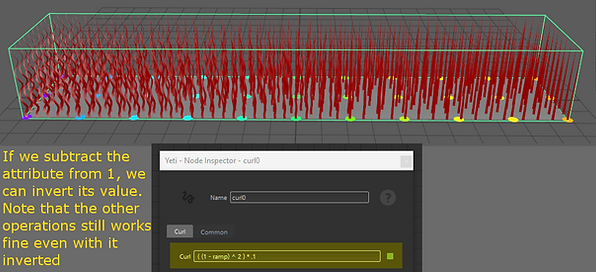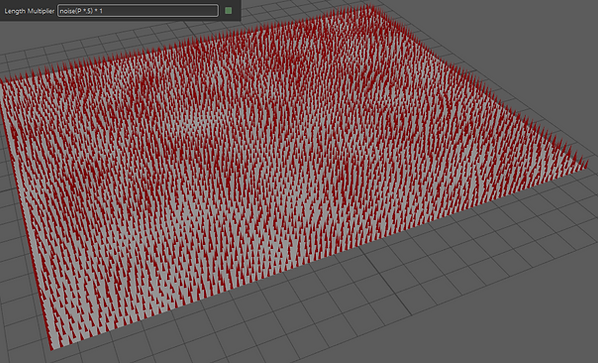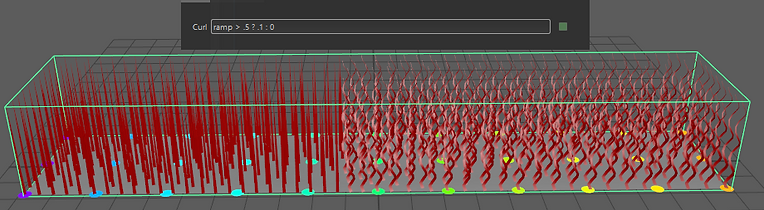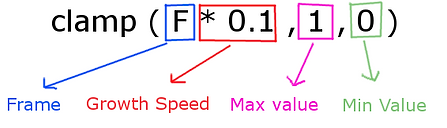top of page

## YETI TUTORIALS

#### ​

Yeti accepts the use of expressions within the fields of its graph nodes. It is a simplified programming language that is based on expressions or arithmetic functions. It is very useful for several cases, or even to speed up tasks. Yeti's own documentation is a good source for querying to use these expressions: http://documentation.peregrinelabs.com/yeti/expressionref.htm

##### Simple Arithmetic Expressions

We can combine attributes with mathematical operations such as addition, multiplication, etc.
The main arithmetic operators are:

-  : Subtraction

*  : Multiplication

/  : Division

^  : Exponential

Parentheses () are essential for grouping arithmetic expressions, since the order of operations can influence the final result. For example, Divisions and Multiplications are performed before Addition and Subtraction, and Exponential is performed before Divisions and Multiplications. Example:

2 + 5 * 3 = 17     First execute 5 * 3 and then + 2
(2 + 5) * 3 = 21     Everything in parentheses is solved first, in this case 2 + 5 = 7, and only then the result is multiplied by 3.

By combining these arithmetic operations with attributes, we have an efficient way of manipulating them within the graph. In the following example, we manipulate a Groom attribute within the Curl node, so that it reaches a different effect without having to edit it within Groom.Example File: Expression_Aritmetics_1.ma

##### Noise Functions

Noise is essential to creating variations on the hairs or instances, creating a more natural look. There are several functions to control them and create different effects. To use a function, consult the Yeti documentation on how it works and always write its name with (). Within these parentheses are the function arguments. In the case of the hash () function it has the following structure:

float hash ( float seed1, [float seed2, ...] )

The first float means that the hash function returns a float at the end of its execution. Its first argument asks for a float value that will be distributed using another float as the seed of the variation.Each fiber has a global attribute called “id”, which has a unique number from zero to how many wires there are. When using it in the hash function, this number is distributed randomly and gains a new unique value between 0 and 1. The second argument changes the seed of the random, that is, it assigns a different value. If there is no second argument, it will be 0 for default.This hash () function is widely used to create variations quickly and efficiently. However, it does not use any standard to distribute its values, and artistic control of this variation may sometimes be necessary. So we can use other types of Noise. Some are exemplified below:

Hash: hash(id)*2SNoise : snoise(P * .4) * 4Noise : noise(P*.5)Cellnoise : cellnoise(P*.5)FBM : fbm(P* .8 , 3.5 , 1 , 1.5 )Turbulence : vturbulence(P, 5, .5, 1)Voronoi : voronoi(P, 1, 1.5, 1.5, 5, 5, 0.5)Example File: Expression_Noise.ma

In these examples, instead of using the id attribute, we use the global variable P, which is the position in the space of each wire. By multiplying P by a greater value, we increase its frequency. Other arguments such as lacunarity and octaves can be used to refine the noise in the functions that allow it.

##### Conditional Expressions

A conditional expression works in this way:

If an statement is true then return command 1, else return command 2

For example, if we have a variable called "var" that has values ​​between 0 and 1, and we want values ​​greater than 0.5 to become 1 and the minor ones become 0:

If "var"> 0.5 then return 1 else return 0

That in the expression language of the Yeti would be:You can use logical operators to perform conditional expressions:

>    Greater than
>=  Greater than or equal to
==  Equal as
<     Less than
<=  Less than or equal to
!=   It is different from

&&  AND
||   ORExample File: Expression_Condition_1.ma

##### Expressions for Animation

We can use expressions to create procedural animations, like fibers growing over time. For this, we use the global variables F (Frame) and time to modify a value.If we use the clamp () function, we can limit the maximum growth value. This function has 3 arguments:Example File: Expression_Animation.ma

bottom of page